# Longest Common Subsequence (LCS)

Given two strings, S1 and S2, the task is to find the length of the Longest Common Subsequence, i.e. longest subsequence present in both of the strings.

A longest common subsequence (LCS) is defined as the longest subsequence which is common in all given input sequences.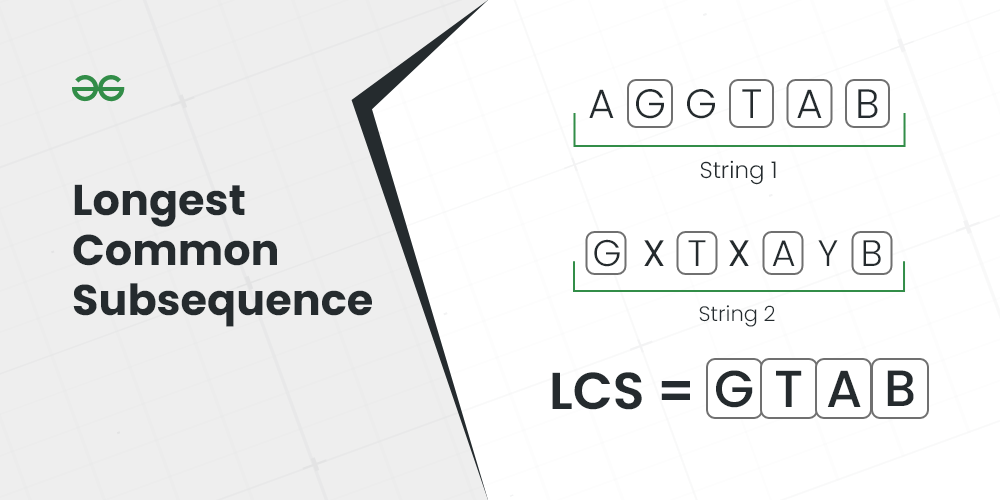Longest Common Subsequence

Examples:

Input: S1 = “AGGTAB”, S2 = “GXTXAYB”
Output: 4
Explanation: The longest subsequence which is present in both strings is “GTAB”.

Input: S1 = “BD”, S2 = “ABCD”
Output: 2
Explanation: The longest subsequence which is present in both strings is “BD”.

Recommended Practice

## Longest Common Subsequence (LCS) using Recursion:

Generate all the possible subsequences and find the longest among them that is present in both strings using recursion.

Follow the below steps to implement the idea:

• Create a recursive function [say lcs()].
• Check the relation between the First characters of the strings that are not yet processed.
• Depending on the relation call the next recursive function as mentioned above.

Below is the implementation of the recursive approach:

## C++

 `// A Naive recursive implementation of LCS problem`   `#include ` `using` `namespace` `std;`   `// Returns length of LCS for X[0..m-1], Y[0..n-1]` `int` `lcs(string X, string Y, ``int` `m, ``int` `n)` `{` `    ``if` `(m == 0 || n == 0)` `        ``return` `0;` `    ``if` `(X[m - 1] == Y[n - 1])` `        ``return` `1 + lcs(X, Y, m - 1, n - 1);` `    ``else` `        ``return` `max(lcs(X, Y, m, n - 1),` `                   ``lcs(X, Y, m - 1, n));` `}`   `// Driver code` `int` `main()` `{` `    ``string S1 = ``"AGGTAB"``;` `    ``string S2 = ``"GXTXAYB"``;` `    ``int` `m = S1.size();` `    ``int` `n = S2.size();`   `    ``cout << ``"Length of LCS is "` `<< lcs(S1, S2, m, n);`   `    ``return` `0;` `}`   `// This code is contributed by rathbhupendra`

## C

 `// A Naive recursive implementation` `// of LCS problem` `#include `   `int` `max(``int` `a, ``int` `b);`   `// Returns length of LCS for X[0..m-1],` `// Y[0..n-1]` `int` `lcs(``char``* X, ``char``* Y, ``int` `i, ``int` `j)` `{` `    ``if` `(X[i] == 0 || Y[j] == 0)` `        ``return` `0;` `    ``if` `(X[i] == Y[j])` `        ``return` `1 + lcs(X, Y, i + 1, j + 1);` `    ``else` `        ``return` `max(lcs(X, Y, i, j + 1),` `                   ``lcs(X, Y, i + 1, j));` `}`   `// Utility function to get max of` `// 2 integers` `int` `max(``int` `a, ``int` `b) { ``return` `(a > b) ? a : b; }`   `// Driver code` `int` `main()` `{` `    ``char` `S1[] = ``"BD"``;` `    ``char` `S2[] = ``"ABCD"``;` `    ``int` `m = ``strlen``(S1);` `    ``int` `n = ``strlen``(S2);` `    ``int` `i = 0, j = 0;`   `    ``// Function Call` `    ``printf``(``"Length of LCS is %d"``, lcs(S1, S2, i, j));`   `    ``return` `0;` `}`

## Java

 `// A Naive recursive implementation of LCS problem in java`   `import` `java.io.*;` `import` `java.util.*;`   `public` `class` `LongestCommonSubsequence {`   `    ``// Returns length of LCS for X[0..m-1], Y[0..n-1]` `    ``int` `lcs(String X, String Y, ``int` `m, ``int` `n)` `    ``{` `        ``if` `(m == ``0` `|| n == ``0``)` `            ``return` `0``;` `        ``if` `(X.charAt(m - ``1``) == Y.charAt(n - ``1``))` `            ``return` `1` `+ lcs(X, Y, m - ``1``, n - ``1``);` `        ``else` `            ``return` `max(lcs(X, Y, m, n - ``1``),` `                       ``lcs(X, Y, m - ``1``, n));` `    ``}`   `    ``// Utility function to get max of 2 integers` `    ``int` `max(``int` `a, ``int` `b) { ``return` `(a > b) ? a : b; }`   `    ``// Driver code` `    ``public` `static` `void` `main(String[] args)` `    ``{` `        ``LongestCommonSubsequence lcs` `            ``= ``new` `LongestCommonSubsequence();` `        ``String S1 = ``"AGGTAB"``;` `        ``String S2 = ``"GXTXAYB"``;` `        ``int` `m = S1.length();` `        ``int` `n = S2.length();`   `        ``System.out.println(``"Length of LCS is"` `                           ``+ ``" "` `+ lcs.lcs(S1, S2, m, n));` `    ``}` `}`   `// This Code is Contributed by Saket Kumar`

## Python3

 `# A Naive recursive Python implementation of LCS problem`     `def` `lcs(X, Y, m, n):` `    ``if` `m ``=``=` `0` `or` `n ``=``=` `0``:` `        ``return` `0` `    ``elif` `X[m``-``1``] ``=``=` `Y[n``-``1``]:` `        ``return` `1` `+` `lcs(X, Y, m``-``1``, n``-``1``)` `    ``else``:` `        ``return` `max``(lcs(X, Y, m, n``-``1``), lcs(X, Y, m``-``1``, n))`     `# Driver code` `if` `__name__ ``=``=` `'__main__'``:` `    ``S1 ``=` `"AGGTAB"` `    ``S2 ``=` `"GXTXAYB"` `    ``print``(``"Length of LCS is"``, lcs(S1, S2, ``len``(S1), ``len``(S2)))`

## C#

 `// C# Naive recursive implementation of LCS problem` `using` `System;`   `class` `GFG {`   `    ``// Returns length of LCS for X[0..m-1], Y[0..n-1]` `    ``static` `int` `lcs(String X, String Y, ``int` `m, ``int` `n)` `    ``{` `        ``if` `(m == 0 || n == 0)` `            ``return` `0;` `        ``if` `(X[m - 1] == Y[n - 1])` `            ``return` `1 + lcs(X, Y, m - 1, n - 1);` `        ``else` `            ``return` `max(lcs(X, Y, m, n - 1),` `                       ``lcs(X, Y, m - 1, n));` `    ``}`   `    ``// Utility function to get max of 2 integers` `    ``static` `int` `max(``int` `a, ``int` `b) { ``return` `(a > b) ? a : b; }`   `    ``// Driver code` `    ``public` `static` `void` `Main()` `    ``{` `        ``String S1 = ``"AGGTAB"``;` `        ``String S2 = ``"GXTXAYB"``;` `        ``int` `m = S1.Length;` `        ``int` `n = S2.Length;`   `        ``Console.Write(``"Length of LCS is"` `                      ``+ ``" "` `+ lcs(S1, S2, m, n));` `    ``}` `}` `// This code is Contributed by Sam007`

## Javascript

 ``

## PHP

 ``

Output

```Length of LCS is 4
```

Time Complexity: O(2m*n)
Auxiliary Space: O(1)

## Longest Common Subsequence (LCS) using Memoization:

If we notice carefully, we can observe that the above recursive solution holds the following two properties:

### 1. Optimal Substructure:

See for solving the structure of L(X[0, 1, . . ., m-1], Y[0, 1, . . . , n-1]) we are taking the help of the substructures of X[0, 1, …, m-2], Y[0, 1,…, n-2], depending on the situation (i.e., using them optimally) to find the solution of the whole.

### 2. Overlapping Subproblems:

If we use the above recursive approach for strings “BD” and “ABCD“, we will get a partial recursion tree as shown below. Here we can see that the subproblem L(“BD”, “ABCD”) is being calculated more than once. If the total tree is considered there will be several such overlapping subproblems.

L(“AXYT”, “AYZX”)
/                                     \
L(“AXY”, “AYZX”)                         L(“AXYT”, “AYZ”)
/                            \                             /                      \
L(“AX”, “AYZX”) L(“AXY”, “AYZ”)   L(“AXY”, “AYZ”)  L(“AXYT”, “AY”)

Approach: Because of the presence of these two properties we can use Dynamic programming or Memoization to solve the problem. Below is the approach for the solution using recursion.

• Create a recursive function. Also create a 2D array to store the result of a unique state.
• During the recursion call, if the same state is called more than once, then we can directly return the answer stored for that state instead of calculating again.

Below is the implementation of the above approach:

## C++

 `// A Top-Down DP implementation` `// of LCS problem` `#include ` `using` `namespace` `std;`   `// Returns length of LCS for X[0..m-1],` `// Y[0..n-1]` `int` `lcs(``char``* X, ``char``* Y, ``int` `m, ``int` `n,` `        ``vector >& dp)` `{` `    ``if` `(m == 0 || n == 0)` `        ``return` `0;` `    ``if` `(X[m - 1] == Y[n - 1])` `        ``return` `dp[m][n] = 1 + lcs(X, Y, m - 1, n - 1, dp);`   `    ``if` `(dp[m][n] != -1) {` `        ``return` `dp[m][n];` `    ``}` `    ``return` `dp[m][n] = max(lcs(X, Y, m, n - 1, dp),` `                          ``lcs(X, Y, m - 1, n, dp));` `}`   `// Driver code` `int` `main()` `{` `    ``char` `X[] = ``"AGGTAB"``;` `    ``char` `Y[] = ``"GXTXAYB"``;`   `    ``int` `m = ``strlen``(X);` `    ``int` `n = ``strlen``(Y);` `    ``vector > dp(m + 1, vector<``int``>(n + 1, -1));` `    ``cout << ``"Length of LCS is "` `<< lcs(X, Y, m, n, dp);`   `    ``return` `0;` `}`

## Java

 `/*package whatever //do not write package name here */` `import` `java.io.*;`   `class` `GFG {`   `    ``// A Top-Down DP implementation of LCS problem`   `    ``// Returns length of LCS for X[0..m-1], Y[0..n-1]` `    ``static` `int` `lcs(String X, String Y, ``int` `m, ``int` `n,` `                   ``int``[][] dp)` `    ``{`   `        ``if` `(m == ``0` `|| n == ``0``)` `            ``return` `0``;`   `        ``if` `(dp[m][n] != -``1``)` `            ``return` `dp[m][n];`   `        ``if` `(X.charAt(m - ``1``) == Y.charAt(n - ``1``)) {` `            ``dp[m][n] = ``1` `+ lcs(X, Y, m - ``1``, n - ``1``, dp);` `            ``return` `dp[m][n];` `        ``}`   `        ``dp[m][n] = Math.max(lcs(X, Y, m, n - ``1``, dp),` `                            ``lcs(X, Y, m - ``1``, n, dp));` `        ``return` `dp[m][n];` `    ``}`   `    ``// Drivers code` `    ``public` `static` `void` `main(String args[])` `    ``{`   `        ``String X = ``"AGGTAB"``;` `        ``String Y = ``"GXTXAYB"``;`   `        ``int` `m = X.length();` `        ``int` `n = Y.length();` `        ``int``[][] dp = ``new` `int``[m + ``1``][n + ``1``];` `        ``for` `(``int` `i = ``0``; i < m + ``1``; i++) {` `            ``for` `(``int` `j = ``0``; j < n + ``1``; j++) {` `                ``dp[i][j] = -``1``;` `            ``}` `        ``}`   `        ``System.out.println(``"Length of LCS is "` `                           ``+ lcs(X, Y, m, n, dp));` `    ``}` `}`   `// This code is contributed by shinjanpatra`

## Python3

 `# A Top-Down DP implementation of LCS problem`   `# Returns length of LCS for X[0..m-1], Y[0..n-1]`     `def` `lcs(X, Y, m, n, dp):`   `    ``if` `(m ``=``=` `0` `or` `n ``=``=` `0``):` `        ``return` `0`   `    ``if` `(dp[m][n] !``=` `-``1``):` `        ``return` `dp[m][n]`   `    ``if` `X[m ``-` `1``] ``=``=` `Y[n ``-` `1``]:` `        ``dp[m][n] ``=` `1` `+` `lcs(X, Y, m ``-` `1``, n ``-` `1``, dp)` `        ``return` `dp[m][n]`   `    ``dp[m][n] ``=` `max``(lcs(X, Y, m, n ``-` `1``, dp), lcs(X, Y, m ``-` `1``, n, dp))` `    ``return` `dp[m][n]`   `# Driver code`     `X ``=` `"AGGTAB"` `Y ``=` `"GXTXAYB"`   `m ``=` `len``(X)` `n ``=` `len``(Y)` `dp ``=` `[[``-``1` `for` `i ``in` `range``(n ``+` `1``)]``for` `j ``in` `range``(m ``+` `1``)]`   `print``(f``"Length of LCS is {lcs(X, Y, m, n, dp)}"``)`   `# This code is contributed by shinjanpatra`

## C#

 `/* C# Naive recursive implementation of LCS problem */` `using` `System;`   `class` `GFG {`   `    ``/* Returns length of LCS for X[0..m-1], Y[0..n-1] */` `    ``static` `int` `lcs(``char``[] X, ``char``[] Y, ``int` `m, ``int` `n,` `                   ``int``[, ] L)` `    ``{` `        ``if` `(m == 0 || n == 0)` `            ``return` `0;`   `        ``if` `(L[m, n] != -1)` `            ``return` `L[m, n];`   `        ``if` `(X[m - 1] == Y[n - 1]) {` `            ``L[m, n] = 1 + lcs(X, Y, m - 1, n - 1, L);` `            ``return` `L[m, n];` `        ``}`   `        ``L[m, n] = max(lcs(X, Y, m, n - 1, L),` `                      ``lcs(X, Y, m - 1, n, L));` `        ``return` `L[m, n];` `    ``}`   `    ``/* Utility function to get max of 2 integers */` `    ``static` `int` `max(``int` `a, ``int` `b) { ``return` `(a > b) ? a : b; }`   `    ``public` `static` `void` `Main()` `    ``{` `        ``String s1 = ``"AGGTAB"``;` `        ``String s2 = ``"GXTXAYB"``;`   `        ``char``[] X = s1.ToCharArray();` `        ``char``[] Y = s2.ToCharArray();` `        ``int` `m = X.Length;` `        ``int` `n = Y.Length;` `        ``int``[, ] L = ``new` `int``[m + 1, n + 1];` `        ``for` `(``int` `i = 0; i <= m; i++) {` `            ``for` `(``int` `j = 0; j <= n; j++) {` `                ``L[i, j] = -1;` `            ``}` `        ``}` `        ``Console.Write(``"Length of LCS is"` `                      ``+ ``" "` `+ lcs(X, Y, m, n, L));` `    ``}` `}`   `// This code is contributed by akshitsaxenaa09`

## Javascript

 `/* A Top-Down DP implementation of LCS problem */`   `/* Returns length of LCS for X[0..m-1], Y[0..n-1] */` `function` `lcs(X, Y, m, n, dp)` `{` `    ``if` `(m == 0 || n == 0)` `        ``return` `0;` `    ``if` `(X[m - 1] == Y[n - 1])` `        ``return` `dp[m][n] = 1 + lcs(X, Y, m - 1, n - 1, dp);`   `    ``if` `(dp[m][n] != -1) {` `        ``return` `dp[m][n];` `    ``}` `    ``return` `dp[m][n] = Math.max(lcs(X, Y, m, n - 1, dp),` `                          ``lcs(X, Y, m - 1, n, dp));` `}`   `/* Driver code */`   `let X = ``"AGGTAB"``;` `let Y = ``"GXTXAYB"``;`   `let m = X.length;` `let n = Y.length;` `let dp = ``new` `Array(m + 1);` `for``(let i = 0; i < m + 1; i++)` `{` `    ``dp[i] = ``new` `Array(n + 1).fill(-1);` `} ` `console.log(``"Length of LCS is "` `+ lcs(X, Y, m, n, dp));`   `// This code is contributed by shinjanpatra`

Output

```Length of LCS is 4
```

Time Complexity: O(m * n) where m and n are the string lengths.
Auxiliary Space: O(m * n) Here the recursive stack space is ignored.

## Longest Common Subsequence (LCS) using Bottom-Up (Tabulation):

We can use the following steps to implement the dynamic programming approach for LCS.

• Create a 2D array dp[][] with rows and columns equal to the length of each input string plus 1 [the number of rows indicates the indices of S1 and the columns indicate the indices of S2].
• Initialize the first row and column of the dp array to 0.
• Iterate through the rows of the dp array, starting from 1 (say using iterator i).
• For each i, iterate all the columns from j = 1 to n:
• If S1[i-1] is equal to S2[j-1], set the current element of the dp array to the value of the element to (dp[i-1][j-1] + 1).
• Else, set the current element of the dp array to the maximum value of dp[i-1][j] and dp[i][j-1].
• After the nested loops, the last element of the dp array will contain the length of the LCS.

See the below illustration for a better understanding:

Illustration:

Say the strings are S1 = “AGGTAB” and S2 = “GXTXAYB”.

First step: Initially create a 2D matrix (say dp[][]) of  size 8 x 7 whose first row and first column are filled with 0.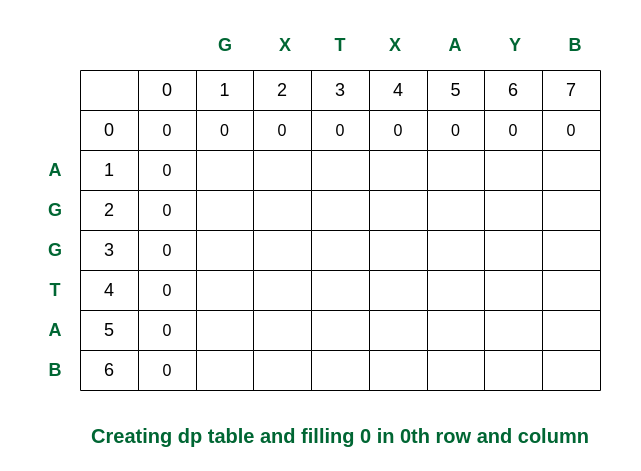Creating the dp table

Second step: Traverse for i = 1. When j becomes 5, S1 and S2 are equal. So the dp[][] is updated. For the other elements take the maximum of dp[i-1][j] and dp[i][j-1]. (In this case, if both values are equal, we have used arrows to the previous rows).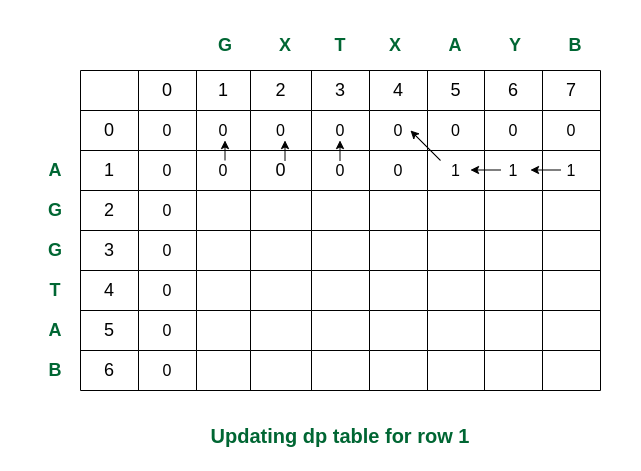Filling the row no 1

Third step: While traversed for i = 2, S1 and S2 are the same (both are ‘G’). So the dp value in that cell is updated. Rest of the elements are updated as per the conditions.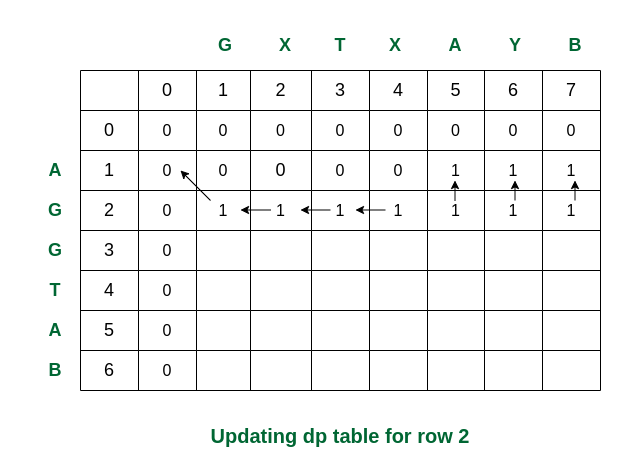Filling the row no. 2

Fourth step: For i = 3, S1 and S2 are again same. The updations are as follows.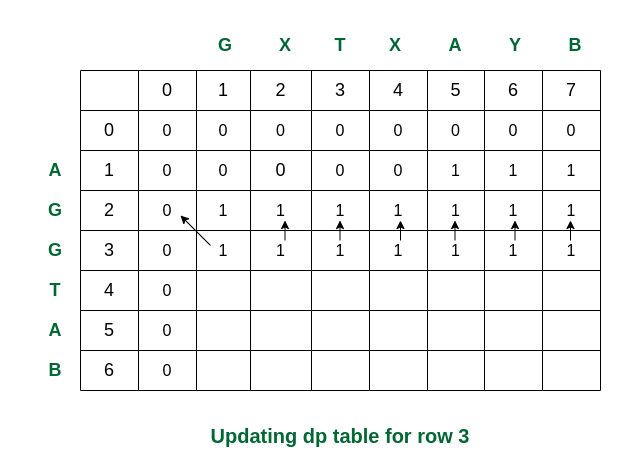Filling row no. 3

Fifth step: For i = 4, we can see that S1 and S2 are same. So dp updated as dp + 1 = 2.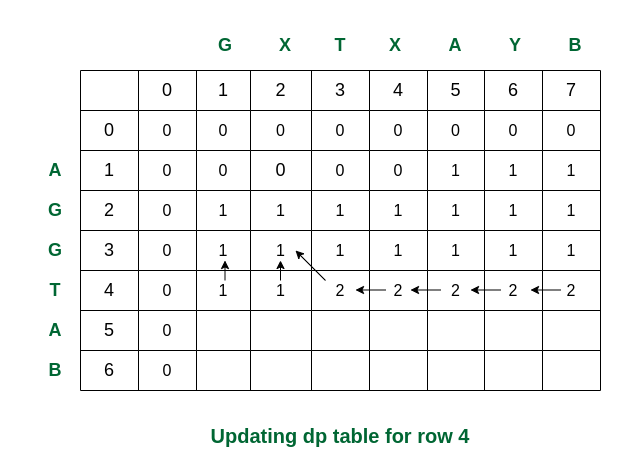Filling row 4

Sixth step: Here we can see that for i = 5 and j = 5 the values of S1 and S2 are same (i.e., both are ‘A’). So dp is updated accordingly and becomes 3.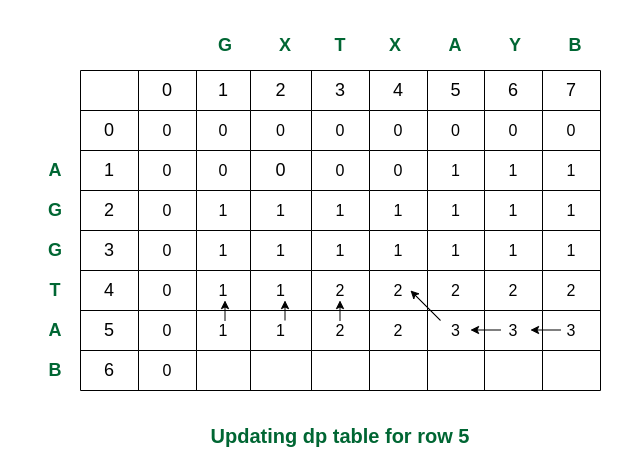Filling row 5

Final step: For i = 6, see the last characters of both strings are same (they are ‘B’). Therefore the value of dp becomes 4.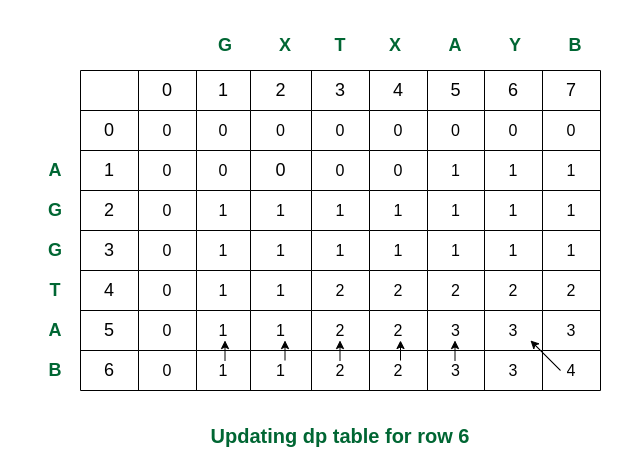Filling the final row

So we get the maximum length of common subsequence as 4.

Following is a tabulated implementation for the LCS problem.

## C++

 `// Dynamic Programming C++ implementation` `// of LCS problem` `#include ` `using` `namespace` `std;`   `// Returns length of LCS for X[0..m-1],` `// Y[0..n-1]` `int` `lcs(string X, string Y, ``int` `m, ``int` `n)` `{`   `    ``// Initializing a matrix of size` `    ``// (m+1)*(n+1)` `    ``int` `L[m + 1][n + 1];`   `    ``// Following steps build L[m+1][n+1]` `    ``// in bottom up fashion. Note that` `    ``// L[i][j] contains length of LCS of` `    ``// X[0..i-1] and Y[0..j-1]` `    ``for` `(``int` `i = 0; i <= m; i++) {` `        ``for` `(``int` `j = 0; j <= n; j++) {` `            ``if` `(i == 0 || j == 0)` `                ``L[i][j] = 0;`   `            ``else` `if` `(X[i - 1] == Y[j - 1])` `                ``L[i][j] = L[i - 1][j - 1] + 1;`   `            ``else` `                ``L[i][j] = max(L[i - 1][j], L[i][j - 1]);` `        ``}` `    ``}`   `    ``// L[m][n] contains length of LCS` `    ``// for X[0..n-1] and Y[0..m-1]` `    ``return` `L[m][n];` `}`   `// Driver code` `int` `main()` `{` `    ``string S1 = ``"AGGTAB"``;` `    ``string S2 = ``"GXTXAYB"``;` `    ``int` `m = S1.size();` `    ``int` `n = S2.size();`   `    ``// Function call` `    ``cout << ``"Length of LCS is "` `<< lcs(S1, S2, m, n);`   `    ``return` `0;` `}`

## Java

 `// Dynamic Programming Java implementation of LCS problem`   `import` `java.util.*;`   `public` `class` `LongestCommonSubsequence {`   `    ``// Returns length of LCS for X[0..m-1], Y[0..n-1]` `    ``int` `lcs(String X, String Y, ``int` `m, ``int` `n)` `    ``{` `        ``int` `L[][] = ``new` `int``[m + ``1``][n + ``1``];`   `        ``// Following steps build L[m+1][n+1] in bottom up` `        ``// fashion. Note that L[i][j] contains length of LCS` `        ``// of X[0..i-1] and Y[0..j-1]` `        ``for` `(``int` `i = ``0``; i <= m; i++) {` `            ``for` `(``int` `j = ``0``; j <= n; j++) {` `                ``if` `(i == ``0` `|| j == ``0``)` `                    ``L[i][j] = ``0``;` `                ``else` `if` `(X.charAt(i - ``1``) == Y.charAt(j - ``1``))` `                    ``L[i][j] = L[i - ``1``][j - ``1``] + ``1``;` `                ``else` `                    ``L[i][j] = max(L[i - ``1``][j], L[i][j - ``1``]);` `            ``}` `        ``}` `        ``return` `L[m][n];` `    ``}`   `    ``// Utility function to get max of 2 integers` `    ``int` `max(``int` `a, ``int` `b) { ``return` `(a > b) ? a : b; }`   `    ``public` `static` `void` `main(String[] args)` `    ``{` `        ``LongestCommonSubsequence lcs` `            ``= ``new` `LongestCommonSubsequence();` `        ``String S1 = ``"AGGTAB"``;` `        ``String S2 = ``"GXTXAYB"``;` `        ``int` `m = S1.length();` `        ``int` `n = S2.length();`   `        ``System.out.println(``"Length of LCS is"` `                           ``+ ``" "` `+ lcs.lcs(S1, S2, m, n));` `    ``}` `}`   `// This Code is Contributed by Saket Kumar`

## Python3

 `# Dynamic Programming implementation of LCS problem`     `def` `lcs(X, Y, m, n):`   `    ``# Declaring the array for storing the dp values` `    ``L ``=` `[[``None``]``*``(n``+``1``) ``for` `i ``in` `range``(m``+``1``)]`   `    ``# Following steps build L[m+1][n+1] in bottom up fashion` `    ``# Note: L[i][j] contains length of LCS of X[0..i-1]` `    ``# and Y[0..j-1]` `    ``for` `i ``in` `range``(m``+``1``):` `        ``for` `j ``in` `range``(n``+``1``):` `            ``if` `i ``=``=` `0` `or` `j ``=``=` `0``:` `                ``L[i][j] ``=` `0` `            ``elif` `X[i``-``1``] ``=``=` `Y[j``-``1``]:` `                ``L[i][j] ``=` `L[i``-``1``][j``-``1``]``+``1` `            ``else``:` `                ``L[i][j] ``=` `max``(L[i``-``1``][j], L[i][j``-``1``])`   `    ``# L[m][n] contains the length of LCS of X[0..n-1] & Y[0..m-1]` `    ``return` `L[m][n]`     `# Driver code` `if` `__name__ ``=``=` `'__main__'``:` `    ``S1 ``=` `"AGGTAB"` `    ``S2 ``=` `"GXTXAYB"` `    ``m ``=` `len``(S1)` `    ``n ``=` `len``(S2)` `    ``print``(``"Length of LCS is"``, lcs(S1, S2, m, n))`   `# This code is contributed by Nikhil Kumar Singh(nickzuck_007)`

## C#

 `// Dynamic Programming implementation of LCS problem` `using` `System;`   `class` `GFG {` `    ``// Returns length of LCS for X[0..m-1], Y[0..n-1]` `    ``static` `int` `lcs(String X, String Y, ``int` `m, ``int` `n)` `    ``{` `        ``int``[, ] L = ``new` `int``[m + 1, n + 1];`   `        ``// Following steps build L[m+1][n+1]` `        ``// in bottom up fashion.` `        ``// Note that L[i][j] contains length of` `        ``// LCS of X[0..i-1] and Y[0..j-1]` `        ``for` `(``int` `i = 0; i <= m; i++) {` `            ``for` `(``int` `j = 0; j <= n; j++) {` `                ``if` `(i == 0 || j == 0)` `                    ``L[i, j] = 0;` `                ``else` `if` `(X[i - 1] == Y[j - 1])` `                    ``L[i, j] = L[i - 1, j - 1] + 1;` `                ``else` `                    ``L[i, j] = max(L[i - 1, j], L[i, j - 1]);` `            ``}` `        ``}` `        ``return` `L[m, n];` `    ``}`   `    ``// Utility function to get max of 2 integers` `    ``static` `int` `max(``int` `a, ``int` `b) { ``return` `(a > b) ? a : b; }`   `    ``// Driver code` `    ``public` `static` `void` `Main()` `    ``{` `        ``String S1 = ``"AGGTAB"``;` `        ``String S2 = ``"GXTXAYB"``;` `        ``int` `m = S1.Length;` `        ``int` `n = S2.Length;`   `        ``Console.Write(``"Length of LCS is"` `                      ``+ ``" "` `+ lcs(S1, S2, m, n));` `    ``}` `}` `// This Code is Contributed by Sam007`

## Javascript

 ``

## PHP

 ``

Output

```Length of LCS is 4
```

Time Complexity: O(m * n) which is much better than the worst-case time complexity of Naive Recursive implementation.
Auxiliary Space: O(m * n) because the algorithm uses an array of size (m+1)*(n+1) to store the length of the common substrings.

## Longest Common Subsequence (LCS) using Bottom-Up (Space-Optimization):

• In the above tabulation approach we are using L[i-1][j] and L[i][j] etc, here the L[i-1] will refers to the matrix L’s previous row and L[i] refers to the current row.
• We can do space optimization by using two vectors one is previous and another one is current.
• When the inner for loop exits we are initializing previous equal to current.

Below is the implementation:

## C++

 `// Dynamic Programming C++ implementation` `// of LCS problem` `#include ` `using` `namespace` `std;`   `int` `longestCommonSubsequence(string& text1, string& text2)` `{` `    ``int` `n = text1.size();` `    ``int` `m = text2.size();`   `    ``// initializing 2 vectors of size m` `    ``vector<``int``> prev(m + 1, 0), cur(m + 1, 0);`   `    ``for` `(``int` `idx2 = 0; idx2 < m + 1; idx2++)` `        ``cur[idx2] = 0;`   `    ``for` `(``int` `idx1 = 1; idx1 < n + 1; idx1++) {` `        ``for` `(``int` `idx2 = 1; idx2 < m + 1; idx2++) {` `            ``// if matching` `            ``if` `(text1[idx1 - 1] == text2[idx2 - 1])` `                ``cur[idx2] = 1 + prev[idx2 - 1];`   `            ``// not matching` `            ``else` `                ``cur[idx2]` `                    ``= 0 + max(cur[idx2 - 1], prev[idx2]);` `        ``}` `        ``prev = cur;` `    ``}`   `    ``return` `cur[m];` `}`   `int` `main()` `{` `    ``string S1 = ``"AGGTAB"``;` `    ``string S2 = ``"GXTXAYB"``;`   `    ``// Function call` `    ``cout << ``"Length of LCS is "` `         ``<< longestCommonSubsequence(S1, S2);`   `    ``return` `0;` `}`

## Java

 `// Dynamic Programming Java implementation of LCS problem`   `import` `java.util.Arrays;`   `public` `class` `GFG {`   `    ``public` `static` `int` `longestCommonSubsequence(String text1, String text2) {` `        ``int` `n = text1.length();` `        ``int` `m = text2.length();`   `        ``// Initializing 2 arrays of size m` `        ``int``[] prev = ``new` `int``[m + ``1``];` `        ``int``[] cur = ``new` `int``[m + ``1``];`   `        ``for` `(``int` `idx1 = ``1``; idx1 < n + ``1``; idx1++) {` `            ``for` `(``int` `idx2 = ``1``; idx2 < m + ``1``; idx2++) {` `                ``// If matching` `                ``if` `(text1.charAt(idx1 - ``1``) == text2.charAt(idx2 - ``1``))` `                    ``cur[idx2] = ``1` `+ prev[idx2 - ``1``];` `                ``// Not matching` `                ``else` `                    ``cur[idx2] = Math.max(cur[idx2 - ``1``], prev[idx2]);` `            ``}` `            ``prev = Arrays.copyOf(cur, m + ``1``);` `        ``}`   `        ``return` `cur[m];` `    ``}`   `    ``public` `static` `void` `main(String[] args) {` `        ``String S1 = ``"AGGTAB"``;` `        ``String S2 = ``"GXTXAYB"``;`   `        ``// Function call` `        ``System.out.println(``"Length of LCS is "` `+ longestCommonSubsequence(S1, S2));` `    ``}` `}`

## Python3

 `def` `longestCommonSubsequence(text1, text2):` `    ``n ``=` `len``(text1)` `    ``m ``=` `len``(text2)`   `    ``# Initializing two lists of size m` `    ``prev ``=` `[``0``] ``*` `(m ``+` `1``)` `    ``cur ``=` `[``0``] ``*` `(m ``+` `1``)`   `    ``for` `idx1 ``in` `range``(``1``, n ``+` `1``):` `        ``for` `idx2 ``in` `range``(``1``, m ``+` `1``):` `            ``# If characters are matching` `            ``if` `text1[idx1 ``-` `1``] ``=``=` `text2[idx2 ``-` `1``]:` `                ``cur[idx2] ``=` `1` `+` `prev[idx2 ``-` `1``]` `            ``else``:` `                ``# If characters are not matching` `                ``cur[idx2] ``=` `max``(cur[idx2 ``-` `1``], prev[idx2])`   `        ``prev ``=` `cur.copy()`   `    ``return` `cur[m]`   `if` `__name__ ``=``=` `'__main__'``:` `    ``S1 ``=` `"AGGTAB"` `    ``S2 ``=` `"GXTXAYB"`   `    ``# Function call` `    ``print``(``"Length of LCS is"``, longestCommonSubsequence(S1, S2))` `# This code is contributed by Rishabh Mathur`

## C#

 `using` `System;`   `class` `Program` `{` `    ``static` `int` `LongestCommonSubsequence(``string` `text1, ``string` `text2)` `    ``{` `        ``int` `n = text1.Length;` `        ``int` `m = text2.Length;` `        ``// initializing 2 arrays of size m` `        ``int``[] prev = ``new` `int``[m + 1];` `        ``int``[] cur = ``new` `int``[m + 1];` `        ``for` `(``int` `idx2 = 0; idx2 < m + 1; idx2++)` `            ``cur[idx2] = 0;` `        ``for` `(``int` `idx1 = 1; idx1 < n + 1; idx1++)` `        ``{` `            ``for` `(``int` `idx2 = 1; idx2 < m + 1; idx2++)` `            ``{` `                ``// if matching` `                ``if` `(text1[idx1 - 1] == text2[idx2 - 1])` `                    ``cur[idx2] = 1 + prev[idx2 - 1];` `                ``// not matching` `                ``else` `                    ``cur[idx2] = 0 + Math.Max(cur[idx2 - 1], prev[idx2]);` `            ``}` `            ``prev = cur;` `        ``}` `        ``return` `cur[m];` `    ``}` `    ``static` `void` `Main()` `    ``{` `        ``string` `S1 = ``"AGGTAB"``;` `        ``string` `S2 = ``"GXTXAYB"``;` `        ``// Function call` `        ``Console.WriteLine(``"Length of LCS is "` `+ LongestCommonSubsequence(S1, S2));` `    ``}` `}`

## Javascript

 `function` `longestCommonSubsequence(text1, text2) {` `    ``const n = text1.length;` `    ``const m = text2.length;`   `    ``// Initializing two arrays of size m` `    ``let prev = ``new` `Array(m + 1).fill(0);` `    ``let cur = ``new` `Array(m + 1).fill(0);`   `    ``for` `(let idx2 = 0; idx2 < m + 1; idx2++) {` `        ``cur[idx2] = 0;` `    ``}`   `    ``for` `(let idx1 = 1; idx1 < n + 1; idx1++) {` `        ``for` `(let idx2 = 1; idx2 < m + 1; idx2++) {` `            ``// If characters match` `            ``if` `(text1[idx1 - 1] === text2[idx2 - 1]) {` `                ``cur[idx2] = 1 + prev[idx2 - 1];` `            ``}` `            ``// If characters don't match` `            ``else` `{` `                ``cur[idx2] = Math.max(cur[idx2 - 1], prev[idx2]);` `            ``}` `        ``}` `        ``// Update the 'prev' array` `        ``prev = [...cur];` `    ``}`   `    ``return` `cur[m];` `}`   `// Main function` `function` `main() {` `    ``const S1 = ``"AGGTAB"``;` `    ``const S2 = ``"GXTXAYB"``;`   `    ``// Function call` `    ``console.log(``"Length of LCS is "` `+ longestCommonSubsequence(S1, S2));` `}`   `// Call the main function` `main();`

Output

```Length of LCS is 4
```

Time Complexity: O(m * n), which remains the same.
Auxiliary Space: O(m) because the algorithm uses two arrays of size m.

Feeling lost in the world of random DSA topics, wasting time without progress? It's time for a change! Join our DSA course, where we'll guide you on an exciting journey to master DSA efficiently and on schedule.
Ready to dive in? Explore our Free Demo Content and join our DSA course, trusted by over 100,000 geeks!